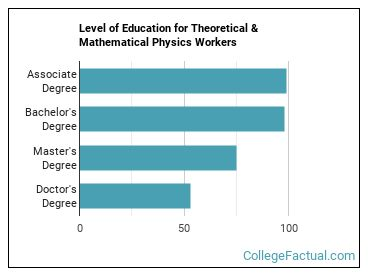by our College Data Analytics Team
Unbiased Factual Guarantee

# 2023 Theoretical & Mathematical Physics Degree Guide

## Rapid Growth in Theoretical & Mathematical Physics Degrees Awarded

#1,257 Most Popular Major
19.0 Degrees Awarded

In 2020-2021, theoretical and mathematical physics was the 1,257 most popular major nationwide with 19 degrees awarded. This represents a 5.3% increase in theoretical and mathematical physics degrees awarded over the prior year's total of 18.

Our 2023 Best Theoretical & Mathematical Physics Schools ranking analyzes 1 of these schools to determine the best overall colleges for theoretical and mathematical physics students. Explore this or one of our many other custom theoretical and mathematical physics rankings further below.

## Best Theoretical & Mathematical Physics Schools by Degree

Bachelor's Degrees in Theoretical & Mathematical Physics

## What Are The Requirements For a Degree in Theoretical & Mathematical Physics

Using scientific rules and methods to solve problems is a required skill for theoretical and mathematical physics majors. Required skills include talking to others to convey information effectively and understanding written sentences and paragraphs in work related documents.

### Theoretical and Mathematical Physics Degree Program Entry Requirements

theoretical and mathematical physics degree applicants generally need have finished high school or their GED. Many schools may also have GPA and SAT/ACT score minimums that must be met. Once you obtain your degree, additional theoretical and mathematical physics certifications required to pursue a career in this field.

### Types of Theoretical & Mathematical Physics Degrees

There are various different levels of theoretical and mathematical physics degrees. You can get anything from a in theoretical and mathematical physics to the highest theoretical and mathematical physics degree, a . The time it takes to complete a theoretical and mathematical physics degree varies depending on the program.

A doctor's degree is the most common level of education achieved by those in careers related to theoretical and mathematical physics, with approximately 34.3% of workers getting one. Find out other typical degree levels for theoretical and mathematical physics workers below.

Most workers in theoretical and mathematical physics have at least a doctor's degree. The chart below shows what degree level those who work in theoretical and mathematical physics have obtained.The education level required is different depending on the theoretical and mathematical physics career you are seeking.

## Theoretical and Mathematical Physics Careers

Below Average Number of Jobs
123k Avg. Related Jobs Salary
11% Growth Job Outlook 2016-26

### Solid Growth Projected for Theoretical and Mathematical Physics Careers

Want a job when you graduate with your theoretical and mathematical physics degree? Theoretical & Mathematical Physics careers are expected to grow 10.8% between 2016 and 2026.

The following options are some of the most in-demand careers related to theoretical and mathematical physics.

### Theoretical and Mathematical Physics Degree Salary Potential

As you might expect, salaries for theoretical and mathematical physics graduates vary depending on the level of education that was acquired.

### Highest Paid Theoretical and Mathematical Physics Careers

Salaries for theoretical and mathematical physics graduates can vary widely by the occupation you choose as well. The following table shows the top highest paying careers theoretical and mathematical physics grads often go into.

## Getting Your Theoretical & Mathematical Physics Degree

With over 9 different theoretical and mathematical physics degree programs to choose from, finding the best fit for you can be a challenge. Fortunately you have come to the right place. We have analyzed all of these schools to come up with hundreds of unbiased theoretical and mathematical physics school rankings to help you with this.

## Top Ranking Lists for Theoretical & Mathematical Physics

One of 8 majors within the Physics area of study, Theoretical & Mathematical Physics has other similar majors worth exploring.

View All Theoretical and Mathematical Physics Related Majors >